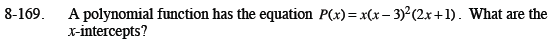Home > CCA2 > Chapter 8 > Lesson 8.3.3 > Problem8-169

8-169.

A polynomial function has the equation P(x) = x(x − 3)2(2x + 1). What are the x-intercepts? Homework Help ✎(0, 0), (3, 0), and (−0.5, 0)